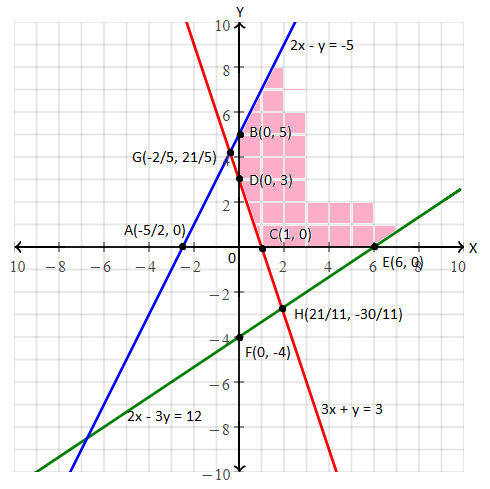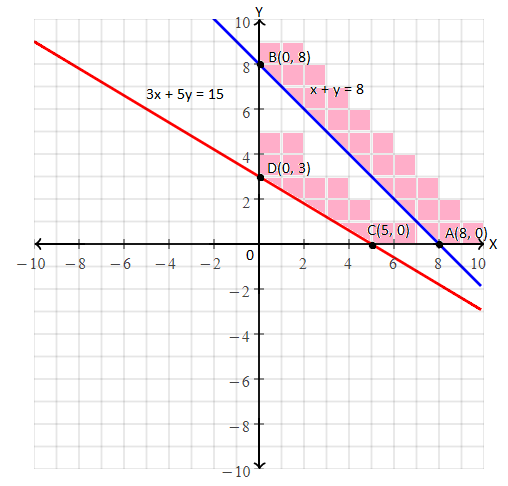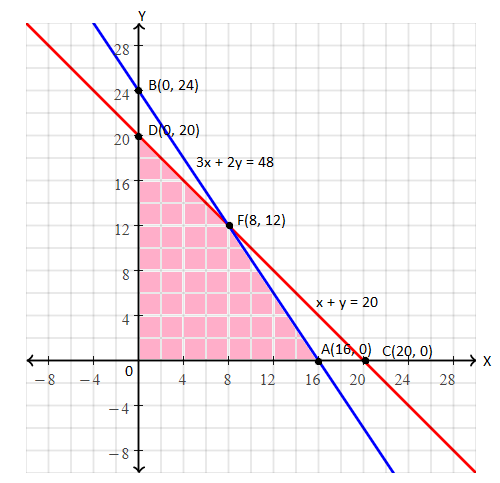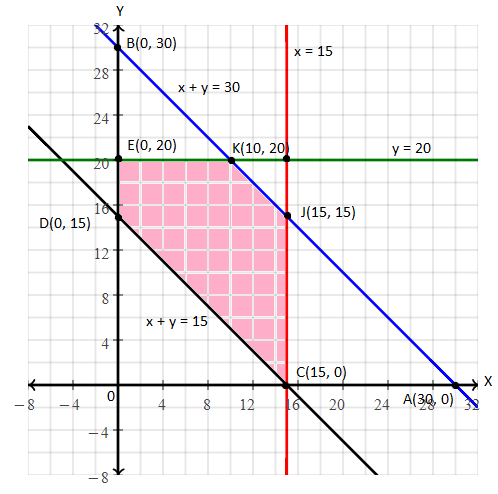# RBSE Maths Class 12 Chapter 15: Linear Programming Important Questions and Solutions

RBSE Class 12 Maths Chapter 15 – Linear Programming Important questions and solutions are given here. The important questions and solutions of this chapter are provided at BYJU’S by the expert faculty. The RBSE Class 12 important questions and solutions available at BYJU’S have complete coverage of all the concepts of Class 12.

Chapter 15 of RBSE Class 12 has only two exercises with the concepts of minimization of cost, maximization of profit and formation of LPP from the given situation. All the questions of this chapter deal with the word problems. In this chapter, students will be taught with the graphical method of solving the LPP along with the formation of LPP.

### RBSE Maths Chapter 15: Exercise 15.1 Textbook Important Questions and Solutions

Question 1: Solve the following linear programming problem graphically.

Minimize Z = -50x + 20y

Subject to the constraints

2x – y ≥ -5

3x + y ≥ 3

2x – 3y ≤ 12

and x ≥ 0, y ≥ 0

Solution:

Converting the given inequalities into equations

2x – y = -5 ….(i)

3x + y = 3 ….(ii)

2x – 3y = 12 ….(iii)

Region represented by 2x – y ≥ – 5, i.e. the line 2x – y = -5 meets the coordinate axes at A(-5/2, 0) and B(0, 5)

Region represented by 3x + y ≥ 3, i.e. the line 3x + y = 3 meets the coordinate axes at C(1, 0) and D(0, 3).

Region represented by 2x – 3y ≤ 12, i.e. the line 2x – 3y = 12 meets the coordinate axes at E(6, 0) and F(0, -4).

Region represented by x ≥ 0, y ≥ 0 is the every point in the first quadrant that satisfies these inequalities.The shaded area is an open and common region which satisfies the given

constraints.

This is the feasible solution of the given linear programming problem.

The value of the given objective function at corner points are given in the below table.

 Corner point Value of Z = -50x + 20y B (0, 5) Z = -50(0) + 20(5) = 100 C (1 , 0) Z = -50(1) + 20(0) = -50 D (0, 3) Z = -50(0) + 20(3) = 60 E (6, 0) Z = -50(6) + 20(0) = -300 Minimum

Minimum value of Z = – 300

Hence, Z is minimum at x = 6 and y = 0.

Question 2: Solve the following linear programming problem graphically.

Maximize

Z = 3x + 2y

Subject to the constraints

x + y ≥ 8

3x + 5y ≤ 15

and x ≥ 0,y ≥ 0

Solution:

Converting the given inequalities into equations

x + y = 8 ….(i)

3x + 5y = 15 ….(ii)

Region represented by x + y ≥ 8, i.e. the line x + y = 8, meets the coordinate axes at A(8, 0) and B(0, 8).

Region represented by 3x + 5y ≤ 15, i.e. the line 3x + 5y = 15 meets the coordinate axes at C(5, 0) and D(0, 3).

Region represented by x ≥ 0, y ≥ 0 is the every point in the first quadrant that satisfies these inequalities.From the graph, it is clear that there is no common feasible region bounded by the two lines.

Therefore, there is no solution that exists for a given objective function.

### RBSE Maths Chapter 15: Exercise 15.2 Textbook Important Questions and Solutions

Question 3: A furniture dealer deals in tables and chairs. He has Rs. 5760 to invest and has a storage space of at most 20 pieces. A table cost Rs. 360 and a chair cost Rs. 240. He estimates that from the sale of one table he can make a profit of Rs. 22 and by selling one chair, he makes a profit of Rs. 18. He wants to know how many tables and chairs he should buy from the available money, so as to maximize his profit, assuming that he can sell all the items which he buys. Solve the optimisation problem graphically.

Solution:

Let x be the number of tables and y be the number of chairs purchased by the dealer.

The cost of x table = Rs. 360x

The cost of y chairs = Rs. 240y

According to the given,

360x + 240 ≤ 5760

And, the place available to have x tables and y chairs is x + y ≤ 20.

The profit earned by dealer on x tables = Rs. 22x

Profit earned on y chairs = Rs. 18y

∴ To earn maximum profits, the objective function is:

Z = 22 x + 18y

Mathematically formulation of LPP is as follows:

Maximum Z = 22x + 18y

Subject to the constraints

360x + 240y ≤ 5760

x + y ≤ 20

x ≥ 0, y ≥ 0

Converting the these inequalities into equations as:

360x + 240y = 5760

⇒ 3x + 2y = 48 ….(i)

and x + y = 20 ….(ii)

Region represented by 3x + 2y ≤ 48, i.e. the line 3x + 2y = 48, meets the coordinate axes at A(16, 0) and B(0, 24).

Region represented by x + y ≤ 20, i.e. the line x + y = 20 meets the coordinate axes at C(20, 0) and D(0, 20).

Region represented by x ≥ 0, y ≥ 0 is the every point in the first quadrant that satisfies these inequalities.The coordinates of the corner points of the feasible region (shaded in the graph) are O(0, 0), A(16, 0), E(8, 12) and D(0, 20).

Here, E(8, 12) is the point of intersection of lines 3x + 2y = 48 and

x + y = 20.

The value of objective function at these points are given in the below table.

 Corner point Value of Z = 22x + 18y O(0, 0) Z = 22(0) + 18(0) = 0 A(16, 0) Z = 22(16) + 18(0) = 352 E(8, 12) Z = 22(8) + 18(12) = 392 Maximum D(0, 20) Z = 22(0) + 18(20) = 360

Therefore, the dealer should purchase 8 tables and 12 chairs to get maximum profit of Rs. 392.

### RBSE Maths Chapter 15: Additional Important Questions and Solutions

Question 1: A brick manufacturer has two depots A and B with a storage capacity of 30,000 and 20,000 bricks. He takes the order from three builders P, Q and R of 15,000, 20,000 and 15,000 numbers of bricks. The cost of transportation to deliver 1000 bricks is given below in the table.

 From/To P Q R A 40 20 30 B 20 60 40

Keeping the transportation cost minimum, how would the manufacturer send the bricks.?

Solution:

Let us assume, Depot A supplies x thousand bricks to builder P and y thousand to builder Q.

And the remaining bricks (30 – x – y) will be supplied to builder R.

Thus, the cost of transportation from builder A to builder P, Q and R are Rs. 40x, Rs. 20y and Rs. 30(30 – x – y) respectively.

From the Depot B the number of bricks supplied to builder P = (15 – x) thousands

Number of bricks supplied to builder Q = (20 – y)

and the number of bricks supplied to builder R = 20 – (15 – x + 20 – y) = (x + y – 15)

Thus, the cost of transportation from depot B to builders P, Q and R are Rs. 20(15 – x), Rs. 60(20 – x) and Rs. 40(x + y – 15).

Hence, the total cost of transportation from both depots A and B to the builders P, Q and R is:

Z = 40x + 20y + 30(30 – x – y) + 20(15 – x) + 60(20 – y) + 40(x + y – 15)

= 30x – 30y + 1800

Mathematical formulation of Linear Programming Problem is:

Minimize Z = 30x – 30y + 1800

Subject to the constraints

x + y ≤ 30

x ≤ 15

y ≤ 20

x + y ≥ 15

x ≥ 0

y ≥ 0

Converting these inequalities into the equations:

x + y = 30 ….(i)

x = 15 ….(ii)

y = 20 ….(iii)

x + y = 15 ….(iv)

x = 0 ….(v)

y = 0 ….(vi)

Region represented by x + y ≤ 30, i.e. the line x + y = 30, meets the coordinate axes at ,A(30, 0) and B(0, 30).

Region represented by x ≤ 15, i.e. the line x = 15 is parallel to the y-axis and its every point will satisfy the inequality in the first quadrant.

Region represented by y ≤ 20, i.e. the line y = 20 is parallel to the x-axis and its every point will satisfy the inequality in the first quadrant.

Region represented by x + y ≥ 15, i.e. the line x + y = 15 meets the coordinate axes at the points C(15, 0) and D(0, 15).

Region represented by x ≥ 0, y ≥ 0 is the every point in the first quadrant that satisfies these inequalities.Thus, the shaded region CJKED (in the graph) represents the feasible solution region of the LPP.

The corner points of this region are C(15, 0), J(15, 15), K(10, 20), E(0, 20) and D(0, 15).

The value of the objective at these corner points is given in the below table.

 Corner point Value of Z = 30x – 30y + 1800 C(15, 0) Z = 30(15) – 30(0) + 1800 = 2250 J(15, 15) Z = 30(15) – 30(15) + 1800 = 1800 K(10, 20) Z = 30(10) – 30(20) + 1800 = 1500 E(0, 20) Z = 30(0) – 30(20) + 1800 = 1200 Minimum D(0, 15) Z = 30(0) – 30(15) + 1800 = 1350

Therefore, from depot A, the builders P, Q and R should be supplied 0, 20000, 10000 number of bricks and from depot B, the builders P, Q and R should be supplied, 15000, 0, 5000 number of bricks.